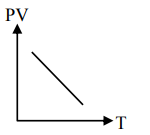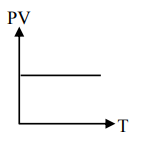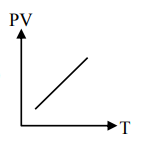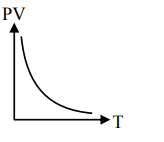# Which of the following graphs represent the behaviorQuestion:

Which of the following graphs represent the behavior of an ideal gas ? Symbols have their usual meaning.

1.2.3.4.Correct Option: , 3

Solution:

$\mathrm{PV}=\mathrm{nRT}$

$P V \propto T$

Straight line with positive slope $(\mathrm{nR})$